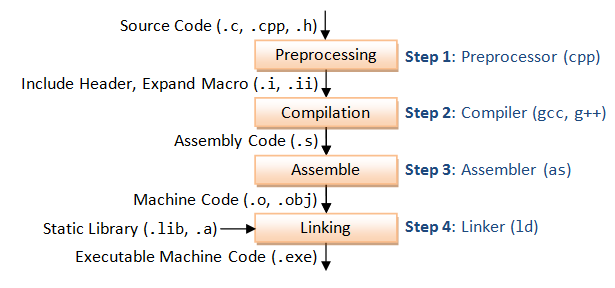??

# C/C++ 中的宏/MacroC/C++ 代碼編譯過程 - 圖片來自 ntu.edu.sg

C/C++ 代碼編譯過程中，可通過相應參數來獲取到各編譯步驟中的產出，比如想看被預處理編譯之后的宏，使用 gcc 使加上 -E 參數。

$gcc -E macro.c ## 宏的定義 通過 #define 指令定義一個宏。 #define NAME_OF_MACRO value 比如，以下代碼定義了一個名為 BUFFER_SIZE 的宏，指代 1024 這個數字。 #define BUFFER_SIZE 1024 使用時， foo = (char *) malloc (BUFFER_SIZE); 使用預處理器編譯： $ gcc -E test.c

foo = (char *) malloc (1024);

### 多行

#include <stdio.h>
#define GREETING_STR \
"hello \
world"
int main() {
printf(GREETING_STR);
return 0;
}

test.c

#include <stdio.h>
#define GREETING_STR \
"hello \
world"
int main() { printf(GREETING_STR); }

int main() {
printf("hello world");
return 0;
}

### 宏展開時的順序

#include <stdio.h>
define GREETING_NAME "wayou"
define GREETING "hello," GREETING_NAME
int main() {
printf(GREETING);
return 0;
}

int main() {
printf("hello," "wayou");
return 0;
}

-#define GREETING_NAME "wayou"
#define GREETING "hello," GREETING_NAME
+#define GREETING_NAME "wayou"

#define BUFSIZE 1020
#define TABLESIZE BUFSIZE
#undef BUFSIZE
#define BUFSIZE 37

## Object-like 宏

Object-like 類型的宏看起來就像普通的數據對象，故名。多用于數字常量的情形下。且宏名一般使用全大寫形式方便識別。像上面示例中，都是 Object-like 的。

## Function-like 宏

#define lang_init()  c_init()
lang_init()
// 編譯后
c_init()

extern void foo(void);
#define foo() /* optimized inline version */
…
foo();
funcptr = foo;

#define lang_init ()    c_init()
lang_init()
// 編譯后：
() c_init()()

## 宏的參數

#define min(X, Y)  ((X) < (Y) ? (X) : (Y))
x = min(a, b);          →  x = ((a) < (b) ? (a) : (b));
y = min(1, 2);          →  y = ((1) < (2) ? (1) : (2));
z = min(a + 28, *p);    →  z = ((a + 28) < (*p) ? (a + 28) : (*p));

### 入參中的括號

macro (array[x = y, x + 1])

### 入參的展開

 min (min (a, b), c)

min (((a) < (b) ? (a) : (b)), (c))

((((a) < (b) ? (a) : (b))) < (c)
? (((a) < (b) ? (a) : (b)))
: (c))

### 參數的缺省

min(, b)        → ((   ) < (b) ? (   ) : (b))
min(a, )        → ((a  ) < ( ) ? (a  ) : ( ))
min(,)          → ((   ) < ( ) ? (   ) : ( ))
min((,),)       → (((,)) < ( ) ? ((,)) : ( ))
min()      error→ macro "min" requires 2 arguments, but only 1 given
min(,,)    error→ macro "min" passed 3 arguments, but takes just 2

### 字符化/Stringizing

#define foo(x) x, "x"
foo(bar)        → bar, "x"

#define WARN_IF(EXP) \
do { if (EXP) \
fprintf (stderr, "Warning: " #EXP "\n"); } \
while (0)
WARN_IF (x == 0);
→ do { if (x == 0)
fprintf (stderr, "Warning: " "x == 0" "\n"); } while (0);

## 拼接

struct command
{
char *name;
void (*function) (void);
};
struct command commands[] =
{
{ "quit", quit_command },
{ "help", help_command },
…
};

#define COMMAND(NAME)  { #NAME, NAME ## _command }
struct command commands[] =
{
COMMAND (quit),
COMMAND (help),
…
};

## 不定參數

#define eprintf(…) fprintf (stderr, __VA_ARGS__)

eprintf ("%s:%d: ", input_file, lineno)
// 編譯后：
fprintf (stderr, "%s:%d: ", input_file, lineno)

C++ 中可這么寫：

#define eprintf(args…) fprintf (stderr, args)

### 不定參數與命名參數混合的情況

#define eprintf(format, …) fprintf (stderr, format, __VA_ARGS__)

## 取消和重置宏

#define FOO 4
x = FOO;        → x = 4;
#undef FOO
x = FOO;        → x = FOO;

### 兩個宏相似的定義

• 類型相同，比如同為對象類型，或函數類型的宏
• 展開后各位置的符號（token）相同
• 如果是函數宏，入參相同
• 空白的不限但出現的位置相同

#define FOUR (2 + 2)
#define FOUR         (2    +    2)
#define FOUR (2 /* two */ + 2)

#define FOUR (2 + 2)
#define FOUR ( 2+2 ) // 空白位置不一樣
#define FOUR (2 * 2) // 宏的內容不一樣
#define FOUR(score,and,seven,years,ago) (2 + 2) // 入參不一樣

## 相關資源

posted @ 2019-06-23 11:53  劉哇勇  閱讀(...)  評論(...編輯  收藏